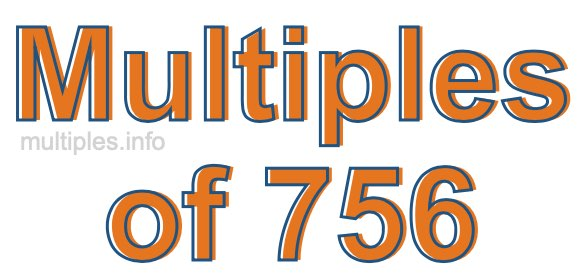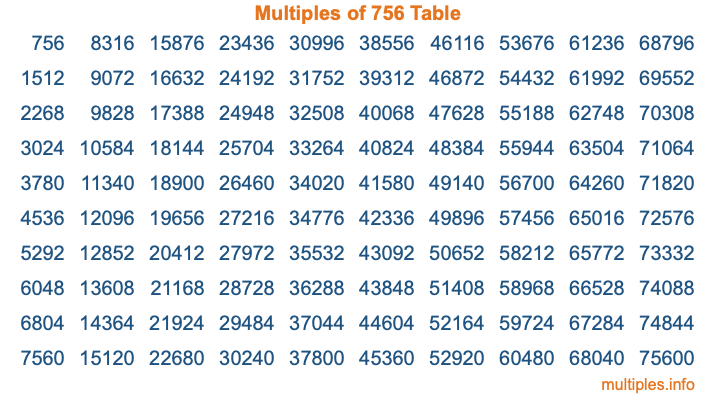Multiples of 756Welcome to the Multiples of 756 page. Here we will first teach you everything you will ever need to know about the multiples of 756, and then give you a study guide summary of everything we taught you to make sure you remember it all. Use this page to look up facts and learn information about the multiples of 756. This page will make you a multiples of seven hundred fifty-six expert!

Definition of Multiples of 756
Multiples of 756 are all the numbers that when divided by 756 equal an integer. Each of the multiples of 756 are called a multiple. A multiple of 756 is created by multiplying 756 by an integer.

Therefore, to create a list of multiples of 756, you start with 1 multiplied by 756, then 2 multiplied by 756, then 3 multiplied by 756, and so on for as long as you want. Thus, the list of the first five multiples of 756 is 756, 1512, 2268, 3024, and 3780. To see a larger list of multiples of 756, see the printable image of Multiples of 756 further down on this page. We also have a category where you can choose any nth multiple of 756.

Multiples of 756 Checker
The Multiples of 756 Checker below checks to see if any number of your choice is a multiple of 756. In other words, it checks to see if there is any number (integer) that when multiplied by 756 will equal your number. To do that, we divide your number by 756. If the the quotient is an integer, then your number is a multiple of 756.

Is  a multiple of 756?

Least Common Multiple of 756 and ...
A Least Common Multiple (LCM) is the lowest multiple that two or more numbers have in common. This is also called the smallest common multiple or lowest common multiple and is useful to know when you are adding our subtracting fractions. Enter one or more numbers below (756 is already entered) to find the LCM.

Check out our LCM Calculator if you need more details about the Least Common Multiple or if you need the LCM for different numbers for adding and subtraction fractions.

nth Multiple of 756
As we stated above, 756 is the first multiple of 756, 1512 is the second multiple of 756, 2268 is the third multiple of 756, and so on. Enter a number below to find the nth multiple of 756.

th multiple of 756

Multiples of 756 vs Factors of 756
756 is a multiple of 756 and a factor of 756, but that is where the similarities end. All postive multiples of 756 are 756 or greater than 756. All positive factors of 756 are 756 or less than 756.

Below is the beginning list of multiples of 756 and the factors of 756 so you can compare:

Multiples of 756: 756, 1512, 2268, 3024, 3780, etc.

Factors of 756: 1, 2, 3, 4, 6, 7, 9, 12, 14, 18, 21, 27, 28, 36, 42, 54, 63, 84, 108, 126, 189, 252, 378, 756

As you can see, the multiples of 756 are all the numbers that you can divide by 756 to get a whole number. The factors of 756, on the other hand, are all the whole numbers that you can multiply by another whole number to get 756.

It's also interesting to note that if a number (x) is a factor of 756, then 756 will also be a multiple of that number (x).

Multiples of 756 vs Divisors of 756
The divisors of 756 are all the integers that 756 can be divided by evenly. Below is a list of the divisors of 756.

Divisors of 756: 1, 2, 3, 4, 6, 7, 9, 12, 14, 18, 21, 27, 28, 36, 42, 54, 63, 84, 108, 126, 189, 252, 378, 756

The interesting thing to note here is that if you take any multiple of 756 and divide it by a divisor of 756, you will see that the quotient is an integer.

Multiples of 756 Table
Below is an image of the first 100 multiples of 756 in a table. The table is in chronological order, column by column. The first column has the first ten multiples of 756, the second column has the next ten multiples of 756, and so on.The Multiples of 756 Table is also referred to as the 756 Times Table or Times Table of 756. You are welcome to print out our table for your studies.

Negative Multiples of 756
Although not often discussed or needed in math, it is worth mentioning that you can make a list of negative multiples of 756 by multiplying 756 by -1, then by -2, then by -3, and so on, to get the following list of negative multiples of 756:

-756, -1512, -2268, -3024, -3780, etc.

Multiples of 756 Summary
Below is a summary of important Multiples of 756 facts that we have discussed on this page. To retain the knowledge on this page, we recommend that you read through the summary and explain to yourself or a study partner why they hold true.

There are an infinite number of multiples of 756.

A multiple of 756 divided by 756 will equal a whole number.

756 divided by a factor of 756 equals a divisor of 756.

The nth multiple of 756 is n times 756.

The largest factor of 756 is equal to the first positive multiple of 756.

756 is a multiple of every factor of 756.

756 is a multiple of 756.

A multiple of 756 divided by a divisor of 756 equals an integer.

756 divided by a divisor of 756 equals a factor of 756.

Any integer times 756 will equal a multiple of 756.

Multiples of a Number
Here you can get the multiples of another number, all with the same attention to detail as we did for multiples of 756 on this page.

Multiples of
Multiples of 757
Did you find our page about multiples of seven hundred fifty-six educational? Do you want more knowledge? Check out the multiples of the next number on our list!